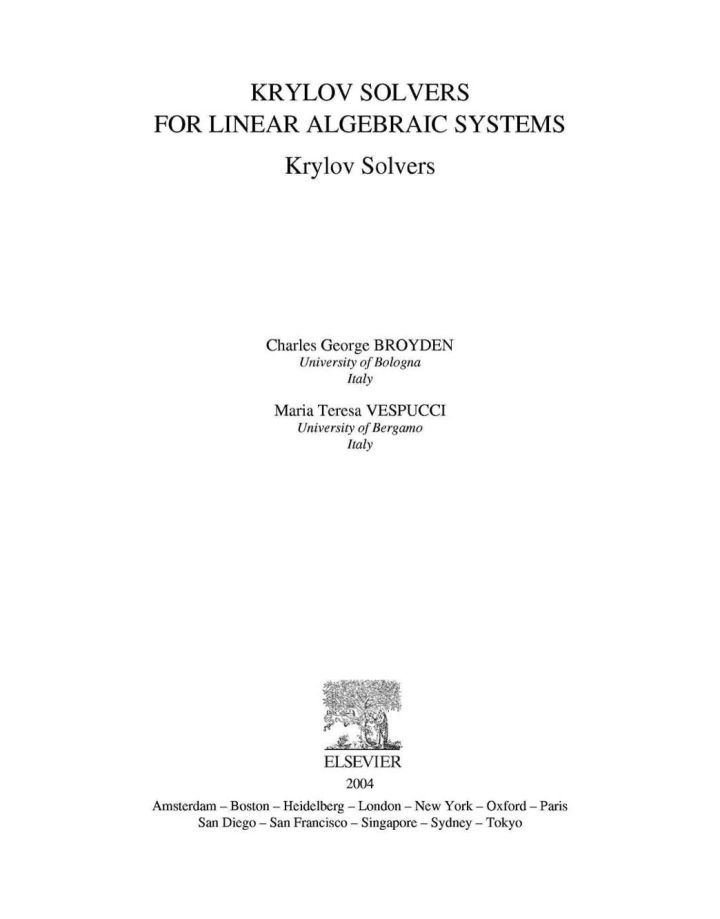# Krylov Solvers for Linear Algebraic Systems: Krylov Solvers

## Krylov Solvers for Linear Algebraic Systems: Krylov Solvers

By Broyden, Charles George; Vespucci, Maria Teresa

### Rent or Buy eTextbook

Expires on Apr 19th, 2022
\$235

Publisher List Price: \$239.99
Savings: \$4.99

The first four chapters of this book give a comprehensive and unified theory of the Krylov methods. Many of these are shown to be particular examples of
the block conjugate-gradient algorithm and it is this observation that
permits the unification of the theory. The two major sub-classes of those
methods, the Lanczos and the Hestenes-Stiefel, are developed in parallel as
natural generalisations of the Orthodir (GCR) and Orthomin algorithms. These
are themselves based on Arnoldi's algorithm and a generalised Gram-Schmidt
algorithm and their properties, in particular their stability properties,
are determined by the two matrices that define the block conjugate-gradient
algorithm. These are the matrix of coefficients and the preconditioning
matrix.

In Chapter 5 the"transpose-free" algorithms based on the conjugate-gradient squared algorithm are presented while Chapter 6 examines the various ways in which the QMR technique has been exploited. Look-ahead methods and general block methods are dealt with in Chapters 7 and 8 while Chapter 9 is devoted to error analysis of two basic algorithms.

In Chapter 10 the results of numerical testing of the more important algorithms in their basic forms (i.e. without look-ahead or preconditioning) are presented and these are related to the structure of the algorithms and the general theory. Graphs illustrating the performances of various algorithm/problem combinations are given via a CD-ROM.

Chapter 11, by far the longest, gives a survey of preconditioning techniques. These range from the old idea of polynomial preconditioning via SOR and ILU preconditioning to methods like SpAI, AInv and the multigrid methods that were developed specifically for use with parallel computers. Chapter 12 is devoted to dual algorithms like Orthores and the reverse algorithms of Hegedus. Finally certain ancillary matters like reduction to Hessenberg form, Chebychev polynomials and the companion matrix are described in a series of appendices.

· comprehensive and unified approach
· up-to-date chapter on preconditioners
· complete theory of stability
· includes dual and reverse methods
· comparison of algorithms on CD-ROM
· objective assessment of algorithms

Subject: Mathematics & Statistics -> Calculus -> Introductory Linear AlgebraPublisher: Elsevier S & T 09/2004
Imprint: Elsevier Science
Language: English
Length: 342 pages

ISBN 10: 0444514740
ISBN 13: 9780444514745
Print ISBN: 9780444514745

Live Chats